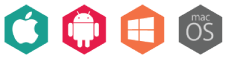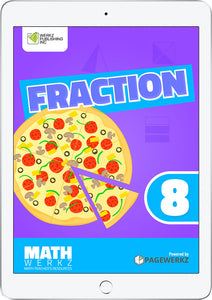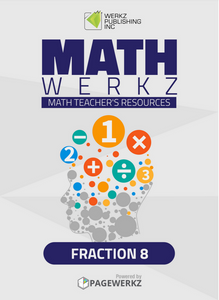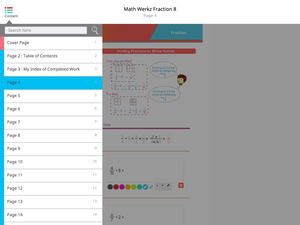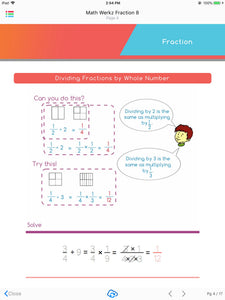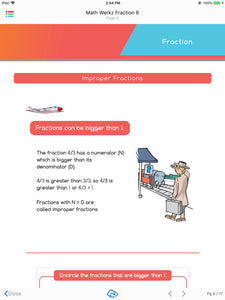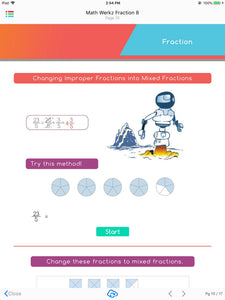# Math Werkz Fraction 8

Regular price \$2.00 Sale

Product Description:

Math Werkz Fraction 8 is a fun, interactive courseware that enables kids to learn about fractions.

Featuring exercises that help parents and teachers facilitate an easier understanding of fractions for their kids and students, Math Werkz Fraction 8 has worksheets about identifying fractions, writing fractions, word problems and creating fraction parts. Among the activities included are dividing fractions by whole number, dividing a fraction by a fraction, changing improper fractions into mixed fractions and vice versa, and word problems. Teachers can also use this as additional exercises or classroom-based activities for their students to work on.

Math Werkz Fraction 8 is one of eight Math Werkz Fraction interactive eBooks.

Math Werkz Series is a Primary Mathematics Teacher Resource consisting of 90+ activity-based worksheets. These worksheets have teacher-enabled tools that can be used to teach and engage students using any available devices like tablets, iPads, smartphones, or classroom devices like whiteboards, SMART Boards or PCs.

Overall, there is a total of 98 Math Werkz interactive eBooks available with topics that range from Factors & Multiples, Fraction, Money, Time, Division, Geometry, Measurement, Graphs and Charts, Multiplication, Percentage, Decimal, Area and Perimeter. Assessment Book, Addition, Subtraction and Number System.

Author: Werkz Publishing Inc.

Number of pages: 17

Available platforms: